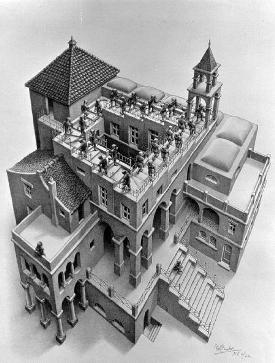# Defacing the Wall

Information Editing the GraphGraph, Wall, Tome Aardvark 27 January 2020 This or Better Link Drive Doc Homepage Invite All Projects

Eric has suggested that the wall should be modified to better fit the needs of the Graph, Wall, Tome Project. There are many extraneous items, for instance the Babylonian calculation of the square root of two and the Archimedes cylinder, there are some redundant items such as the Yang-Mills equations and the Maxwell equations, and the placement of equations on the wall doesn't reflect the groupings of the principles they embody. The Wall should reflect and expand the organization of ideas in the Graph.

## Modification Ideas

What should be in and what should be out of the future wall?
Item In Out Maybe Why?
Klein-Gordon equation:' $$\frac{1}{c^2} \frac{\partial^2}{\partial t^2} \psi - \nabla^2 \psi + \frac{m^2 c^2}{\hbar^2} \psi = 0$$
Lorenz Attractor
Higgs Boson $$V(\phi) = \mu^2\phi^\dagger\phi + \lambda(\phi^\dagger\phi)^2$$
Bianchi identities First identity $$D\Theta = \Omega \wedge \theta$$ Second identity: $$D \Omega = 0$$
Non relativistic Schrödingers equation
Cosmological ‘constant’ added to Einstein field equations
Babylonian computation of the square root of 2
Borel–Weil–Bott theorem
Equiangular spiral drawn in "golden" rectangle (side ratio = golden mean g), ratio of consecutive Fibonacci numbers...
Singer becomes Atiyah Patodi Singer
Einstein GR eqn
Schrodinger eqn
dirac eqn
Atiyah-singer-theorem
Yang Mills eqns
Einstein mass-energy relation
Maxwell’s eqns
Stoke’s thm
Heisenberg uncertainty relation
associtivity in QFT
Yang Baxter eqn
diagram for black hole
cell decomposition torus, gauss-bonnett
aharonov bohm effect
supergrav lagrangian, E8 root diagram
Kepler’s 2nd law
Newtons force-accel
kepler’s 3rd
newton’s grav
defining relation supersymmetry
the boundary of a boundary is zero
euler zeta function
Interaction between two strings
Jones polynomial
regular polyhedra
Euler characteristic
archimedes volume of sphere
Navier stokes
Bochner Weitzenböck formula
Chern–Weil homomorphism
Quillen connection on the determinant line bundle
Bott periodicity
KO-Theory
K-Theory
Tits-Freudenthal Magic Square
formula for the deformation complex
formula relating Lie, covariant, and exterior derivatives
fundamental theory of Riemannian geometry, done right
recipe for Hamiltonian dynamics
something involving the Pfaffian and Euler class
decomposition of the Riemann curvature tensor
position and momentum operators in geometric quantization
Witten’s morse complex
Fierz identity
Noether's theorem
Dynkin diagrams
Hurewicz homomorphism
Leray-Serre spectral sequence
Torsion tensor
Variation of Action is 0 $$\delta S = 0$$ Everything stems from here.

## Archived Material

Cam's Expanded Wall Proposal
• A modified and annotated version of the wall is available here. More information can be found on the corresponding subproject page.
• The code for the modified wall animation is available on Github.

Minimalistic Wall Proposal

The following modified version of the Wall only contains equations and figures that are directly relevant for the graph.